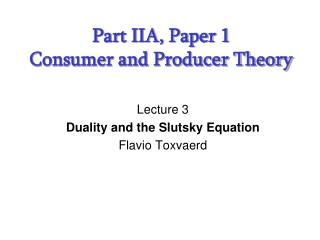# Part IIA, Paper 1 Consumer and Producer Theory - PowerPoint PPT PresentationDownload PresentationPart IIA, Paper 1 Consumer and Producer Theory

Presentation Description
Download Presentation## Part IIA, Paper 1 Consumer and Producer Theory

- - - - - - - - - - - - - - - - - - - - - - - - - - - E N D - - - - - - - - - - - - - - - - - - - - - - - - - - -
##### Presentation Transcript

1. Part IIA, Paper 1Consumer and Producer Theory Lecture 3 Duality and the Slutsky Equation Flavio Toxvaerd

2. Today’s Outline • Dual approaches to consumer’s problem • Hicksian demand function • Expenditure function • Shephard’s lemma • Slutsky equation • The big picture (various relations)

3. Recap Solve Marshallian demand x1(p,m) and x2(p,m) Substitute into u(x,y) Roy’s identity Indirect utility v(p,m)

4. The Envelope Theorem

5. The Envelope Theorem

6. Application 1 (again…) Marginal utility of Income The marginal utility of income is given by the Lagrange multiplier

7. Application 2 (again…) Roy’s Identity Roy’s Identity

8. Duality Primal Problem Dual Problem x2 x2 x1 x2

9. Dual Problem The dual problem gives us the minimum expenditure required for the consumer to achieve a particular level of utility. First Order Conditions

10. Dual Problem Eliminating the Lagrange multiplier () gives MRS = Ratio of Prices Slope of Indifference Curve = Slope of Budget Line Utility Constraint

11. Hicksian Demand Function • Solving these two equations, gives the levels of demand which achieve the desired utility level at the lowest cost • When indifference curves are strictly convex the solution is unique and we may write demand as a function of prices and the desired utility level These are called Hicksian/Compensated Demand Functions

12. x2 Hicksian Demand Function u x1 p1 Hicksian (Compensated) Demand Curve p1 (Hicksian Demand) = Substitution Effect p’1 x1 h1(p1,p2,m) h1(p’1,p2,m)

13. Expenditure Function We can now determine the minimum expenditure required to achieve a specific utility level, given the prices:

14. Properties of the Expenditure Function • Homogeneous of degree 1 in prices • Proof: No change in relative prices, so no change in optimal solution - so expenditure changes by same proportion. • Concave in prices • Proof: If prices change, expenditure will increase linearly if consumption bundle unchanged. Thus any change in consumption reduces expenditure - and expenditure increases less than linearly. • Increasing in prices if u(x) is an increasing function

15. Shephard’s Lemma Shephard’s Lemma

16. Passive Expenditure Indirect Effect Actual Expenditure (1) Will notdo any worse than “Passive Expenditure” (2) As change in Px goes to zero, indirect effect becomes irrelevant because original choice very close to optimal

17. Indirect Utility and Expenditure Functions • Recall: Indirect utility function gave us the level of utility given prices and income • Expenditure function gives us level of income required to achieve a specified utility level • Thus the indirect utility function is just the inverse of the expenditure function - and vice versa

18. Duality in Demand Functions u0 x2 e0 x1 So, we can write

19. Slutsky Equation We have shown that Slutsky Equation Income Effect Substitution Effect

20. Primal Approach Dual Approach Duality Integrability problem Solve Solve Marshallian Demand x1(p,m) and x2(p,m) Hicksian Demand Equivalent if Substitute into cost equation Roy’s Identity Substitute into u(x,y) Shephard’s Lemma Indirect Utility v(p,m) Invert Expenditure Function

21. Integrability Problem • The problem with all of this is that the utility function is not observable - only behaviour (demand) is observable • Thus we are in danger of having a wonderfully elegant theory of consumer behaviour - which relies on an unobservable function! • The Slutsky equation is defined for every price/commodity combination

22. Integrability Problem • The ‘nice’ assumption of convexity and more is better on the utility function impose restrictions on the nature of the matrix of Slutsky equations • These conditions turn out to be both necessary and sufficient for the demand function to be generated by a utility function which possesses these ‘nice’ properties. • Thus we can now identify precisely whether or not our theoretical framework is appropriate

23. Summary • Can approach consumer’s problem from different angles • Having one function, may recover other functions

24. Readings • Varian, Microeconomic Analysis, chapters 6, 7, 8

25. Next Time… • Revealed preferences • WARP, SARP, GARP • Indices